Finding second order derivatives- Implicit form

Chapter 5 Class 12 Continuity and Differentiability
Concept wise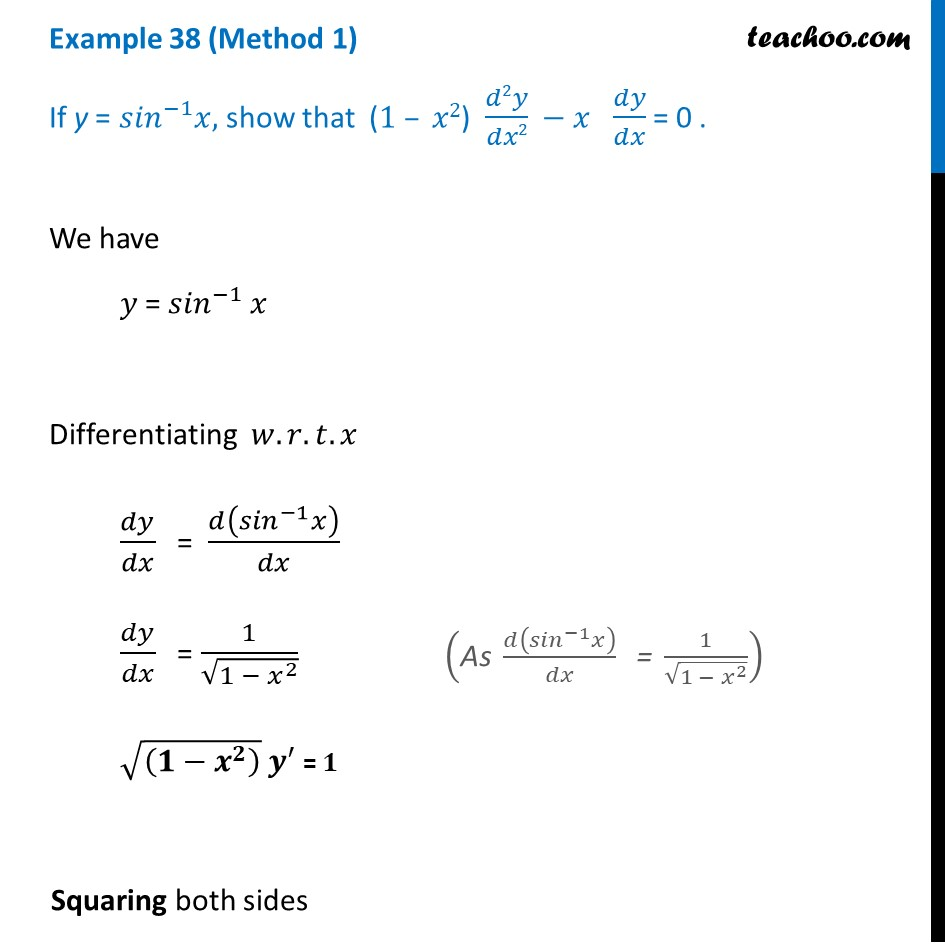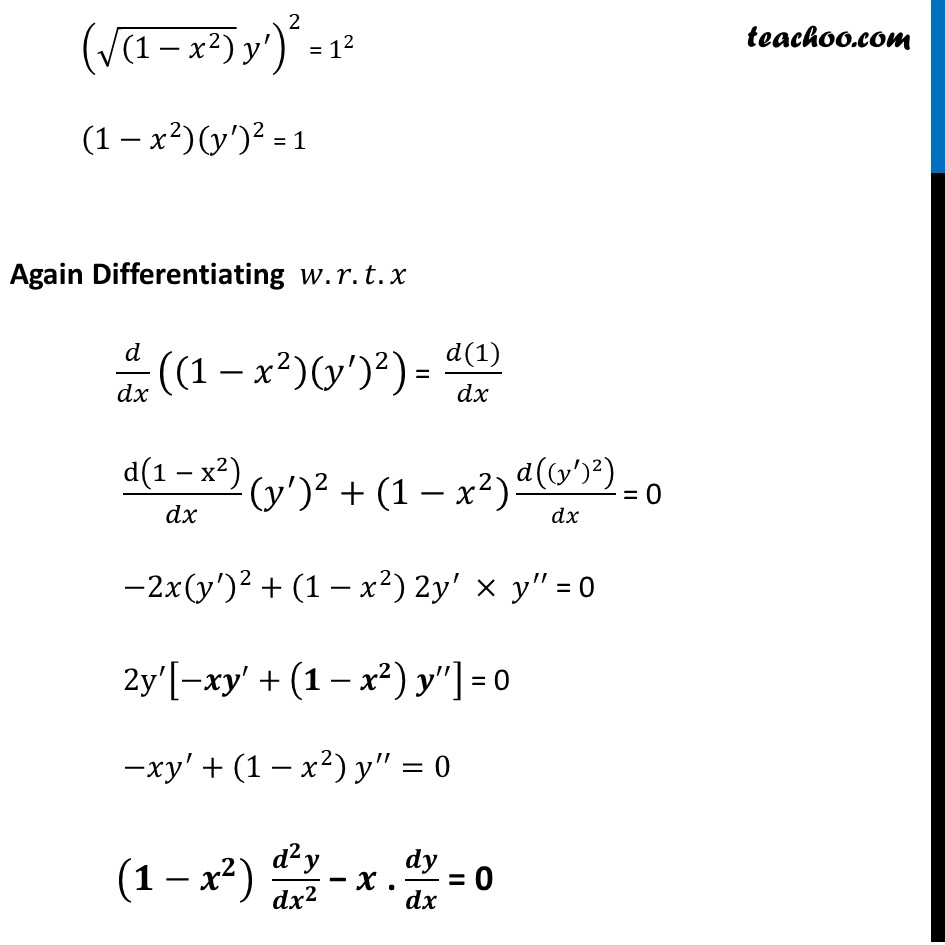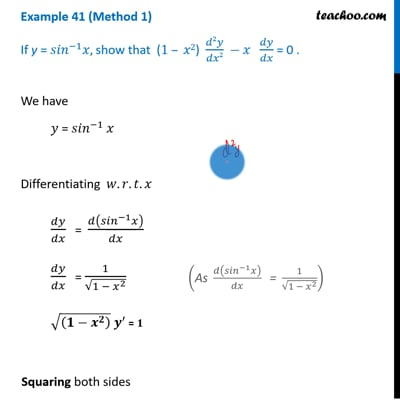This video is only available for Teachoo black users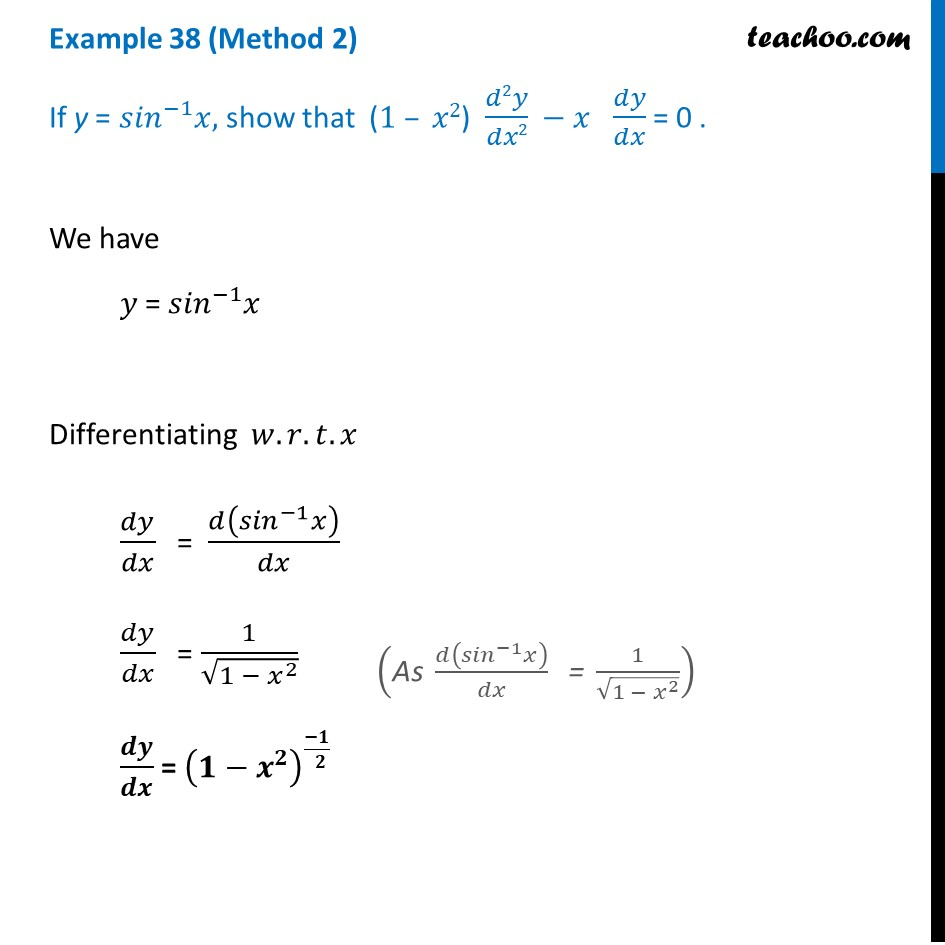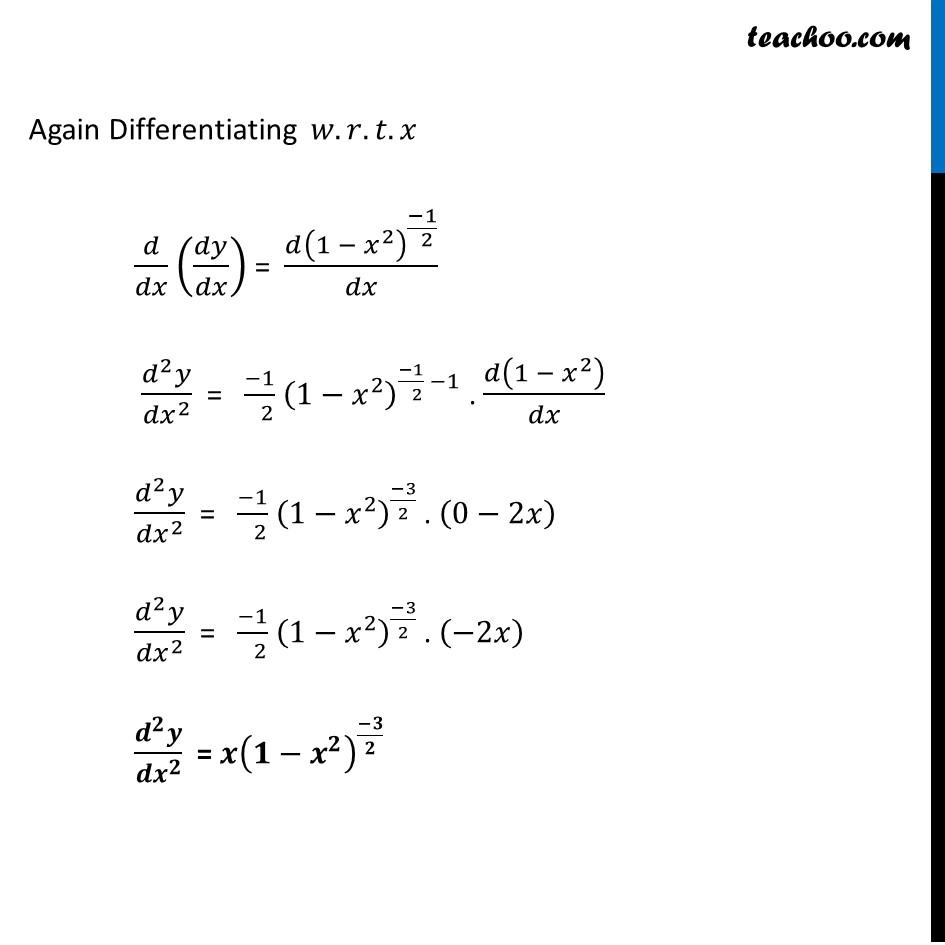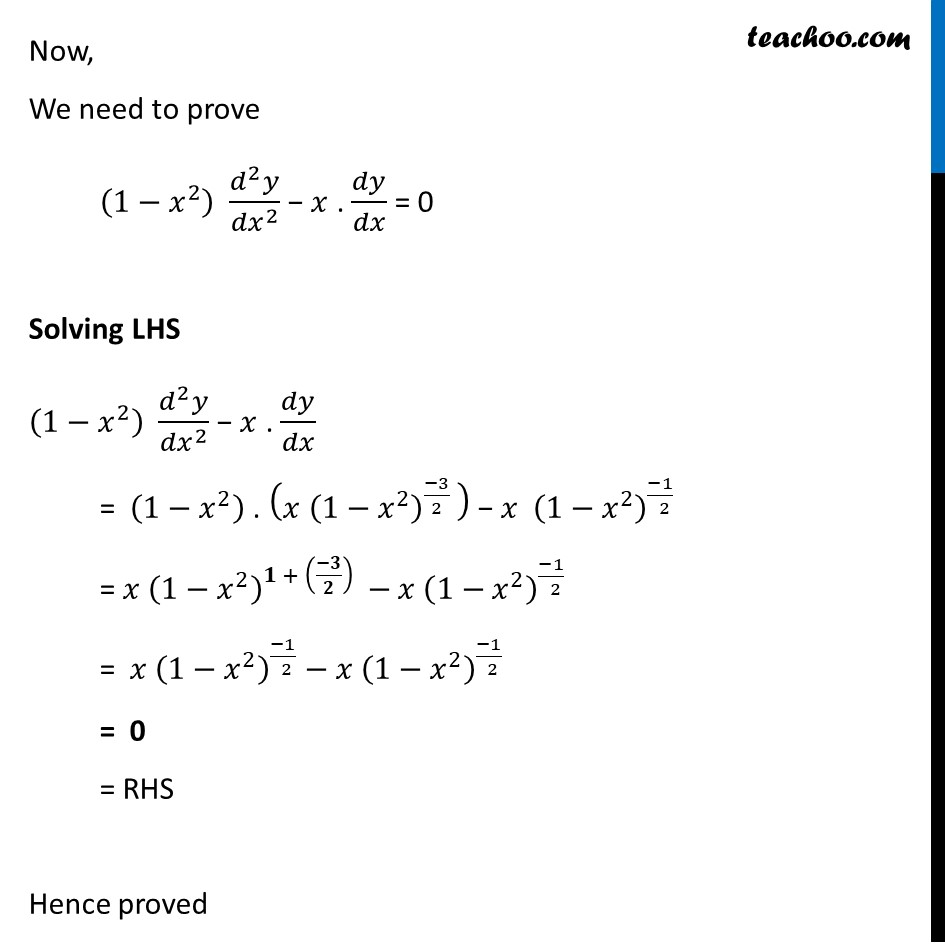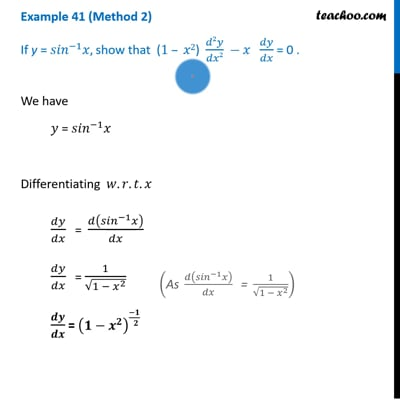This video is only available for Teachoo black users

Learn in your speed, with individual attention - Teachoo Maths 1-on-1 Class

### Transcript

Example 38 (Method 1) If y = 〖𝑠𝑖𝑛〗^(−1) 𝑥, show that (1 – 𝑥2) 𝑑2𝑦/𝑑𝑥2 − 𝑥 𝑑𝑦/𝑑𝑥 = 0 . We have 𝑦 = 〖𝑠𝑖𝑛〗^(−1) 𝑥 Differentiating 𝑤.𝑟.𝑡.𝑥 𝑑𝑦/𝑑𝑥 = 𝑑(〖𝑠𝑖𝑛〗^(−1) 𝑥)/𝑑𝑥 𝑑𝑦/𝑑𝑥 = 1/√(〖1 − 𝑥〗^2 ) √((𝟏−𝒙^𝟐 ) ) 𝒚^′ = 𝟏 Squaring both sides ("As " 𝑑(〖𝑠𝑖𝑛〗^(−1) 𝑥)/𝑑𝑥 " = " 1/√(〖1 − 𝑥〗^2 )) (√((1−𝑥^2 ) ) 𝑦^′ )^2 = 1^2 (1−𝑥^2 )(𝑦^′ )^2 = 1 Again Differentiating 𝑤.𝑟.𝑡.𝑥 𝑑/𝑑𝑥 ((1−𝑥^2 )(𝑦^′ )^2 ) = (𝑑(1))/𝑑𝑥 d(1 − x^2 )/𝑑𝑥 (𝑦^′ )^2+(1−𝑥^2 ) 𝑑((𝑦^′ )^2 )/𝑑𝑥 = 0 −2𝑥(𝑦^′ )^2+(1−𝑥^2 ) 2𝑦^′ × 𝑦^′′ = 0 〖2y〗^′ [−𝒙𝒚^′+(𝟏−𝒙^𝟐 ) 𝒚^′′ ] = 0 −𝑥𝑦^′+(1−𝑥^2 ) 𝑦^′′=0 (〖𝟏−𝒙〗^𝟐 ) (𝒅^𝟐 𝒚)/〖𝒅𝒙〗^𝟐 − 𝒙 . 𝒅𝒚/𝒅𝒙 = 0 Example 38 (Method 2) If y = 〖𝑠𝑖𝑛〗^(−1) 𝑥, show that (1 – 𝑥2) 𝑑2𝑦/𝑑𝑥2 − 𝑥 𝑑𝑦/𝑑𝑥 = 0 . We have 𝑦 = 〖𝑠𝑖𝑛〗^(−1) 𝑥 Differentiating 𝑤.𝑟.𝑡.𝑥 𝑑𝑦/𝑑𝑥 = 𝑑(〖𝑠𝑖𝑛〗^(−1) 𝑥)/𝑑𝑥 𝑑𝑦/𝑑𝑥 = 1/√(〖1 − 𝑥〗^2 ) 𝒅𝒚/𝒅𝒙 = (〖𝟏−𝒙〗^𝟐 )^((−𝟏)/( 𝟐)) ("As " 𝑑(〖𝑠𝑖𝑛〗^(−1) 𝑥)/𝑑𝑥 " = " 1/√(〖1 − 𝑥〗^2 )) Again Differentiating 𝑤.𝑟.𝑡.𝑥 𝑑/𝑑𝑥 (𝑑𝑦/𝑑𝑥) = (𝑑(〖1 − 𝑥〗^2 )^((−1)/( 2)))/𝑑𝑥 (𝑑^2 𝑦)/〖𝑑𝑥〗^2 = (−1)/( 2) (〖1−𝑥〗^2 )^((−1)/( 2) −1) . 𝑑(〖1 − 𝑥〗^2 )/𝑑𝑥 (𝑑^2 𝑦)/〖𝑑𝑥〗^2 = (−1)/( 2) (〖1−𝑥〗^2 )^((−3)/2 ). (0−2𝑥) (𝑑^2 𝑦)/〖𝑑𝑥〗^2 = (−1)/( 2) (〖1−𝑥〗^2 )^((−3)/2 ). (−2𝑥) (𝒅^𝟐 𝒚)/〖𝒅𝒙〗^𝟐 = 𝒙(〖𝟏−𝒙〗^𝟐 )^((−𝟑)/𝟐 ) Now, We need to prove (〖1−𝑥〗^2 ) (𝑑^2 𝑦)/〖𝑑𝑥〗^2 − 𝑥 . 𝑑𝑦/𝑑𝑥 = 0 Solving LHS (〖1−𝑥〗^2 ) (𝑑^2 𝑦)/〖𝑑𝑥〗^2 − 𝑥 . 𝑑𝑦/𝑑𝑥 = (〖1−𝑥〗^2 ) . (𝑥〖 (〖1−𝑥〗^2 )〗^((−3)/2 ) ) − 𝑥 (〖1−𝑥〗^2 )^((−1)/( 2)) = 𝑥〖 (〖1−𝑥〗^2 )〗^(𝟏 + ((−𝟑)/𝟐) )−𝑥 (〖1−𝑥〗^2 )^((−1)/( 2)) = 𝑥〖 (〖1−𝑥〗^2 )〗^((−1)/( 2))−𝑥 (〖1−𝑥〗^2 )^((−1)/( 2)) = 0 = RHS Hence proved